Multivariable Calculus : Arc Length

Example Questions

Example Question #1 : Partial Differentiation

Determine the length of the curve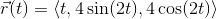, on the interval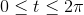.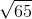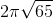Explanation:

First we need to find the tangent vector, and find its magnitude.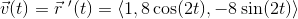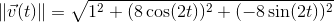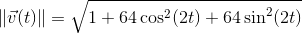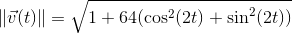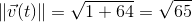Now we can set up our arc length integral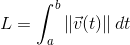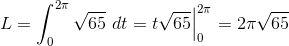Example Question #2 : Partial Differentiation

Determine the length of the curve, on the interval.Explanation:

First we need to find the tangent vector, and find its magnitude.Now we can set up our arc length integral